# What's Battery Current and Motor Current exactly ? What's their difference?

Many people are confused of the concept of battery current and motor current, what's exactly they are? and what's the difference. Here comes detail explanations.The relationship between power &voltage &current: P=UI, U is voltage, I is current, if the voltage keep the same, the power is become bigger as the current become bigger.

The relationship between the rated power& rated voltage &rated current of motor: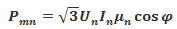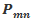is rated power，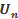is rated voltage，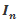is rated current，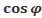is rated power factor，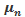is electrical efficiency.

Whenkeep equal,is inversely proportional to, then what relationship is between rotor speed and current of motor based on the above statement.

Inductive electromotive force formula for motors: E=-K , K is constant of proportionality, Φis magnetic flux，t is time.

Inductive electromotive force is the essence of electromagnetic induction. Inductive current in the magnetic field will be ampere force, resulting in magnetic moment, thus driving the coil rotation.

From the above formula, we know that the electromotive force is proportional to the magnetic flux.

The formula for calculating magnetic flux φ=b*s*cosθ, When the plane with an area of s is perpendicular to the direction of the magnetic field, the angular θ is 0, the cosθ is equal to 1, and the formula becomes aΦ=B*S.Without load: when the motor start-up, on this moment the rotor speed is 0, and the coil winding is almost connected directly to the positive and negative poles of the power supply, and the current passing through the coil is very large. According to ohm’s law, U=IR，I=U/R, and the resistance of copper wire is small, so the instantaneous current is very large, of course this is only a transient. Normally the rotor will rotate quickly, and the “induced electromotive force” will be generated between the rotor and the stator. As the motor speed increases, the “induced electromotive force” will increase rapidly until a certain limit value-supply voltage, the inductive electromotive force is opposite to the supply voltage, so the current loaded on the coil will be small.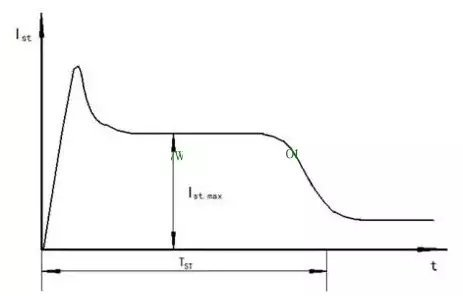Load: when we add load on the motor, such as loading on the electric skateboard, as the speed of the motor decreases, the “induced electromotive force” decreases and the relative voltage increases, then the coil current increases. The increase of current will increase the rotor speed, then current decreases until a dynamitic balance is reached.

In general, the faster the motor speed, the smaller the current; the slower the motor speed, the greater the current. Current, voltage is a dynamic balancing process.

When setting the maximum current parameters of the motor, we should confirm the rated current of the motor. If the set maximum current exceeds the rated current of the motor, the motor maybe burn out, so we should generally set the parameter according to the rated current.

When setting the maximum current parameter of the battery, we should confirm the rated current of the controller. If the maximum current is set to exceed the rated current of the control, the controller maybe burn out.

When these two parameters are set, the rated power of the motor and the power of the controller should be combined, If the output power of the controller is greater than the rated power of the motor, the motor may burn out.

Why is the current parameter negative number in vesc tool?

Vesc use regenerative braking that will cause the motor to produce inductive current, and this inductive current will feedback to battery and charge the battery, so the direction of this current is opposite to the direction of the current running by the motor driving load, then it appears negative number.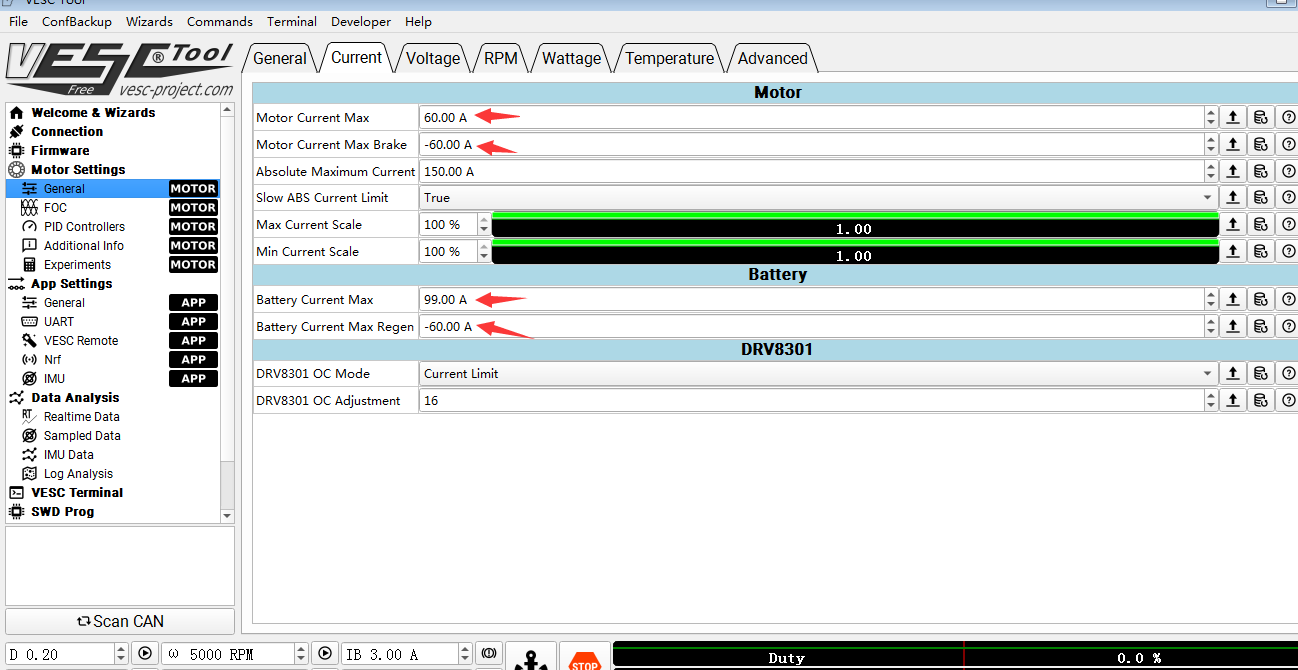What max current that I can set up for Ubox?

We suggest the max limitation setting for Ubox is: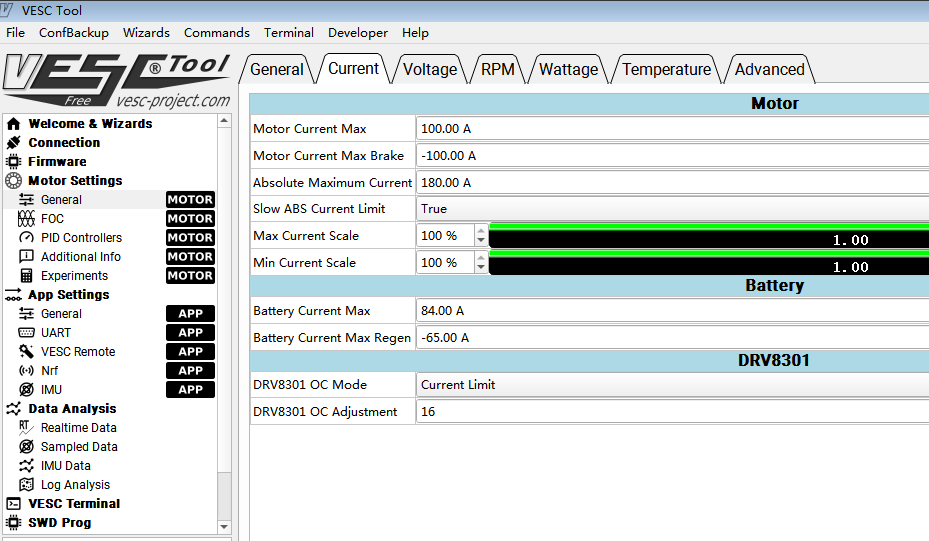And this is NOT fit for all kinds of customer's needs. Please set parameter based on your actual battery and motor spec conditions.